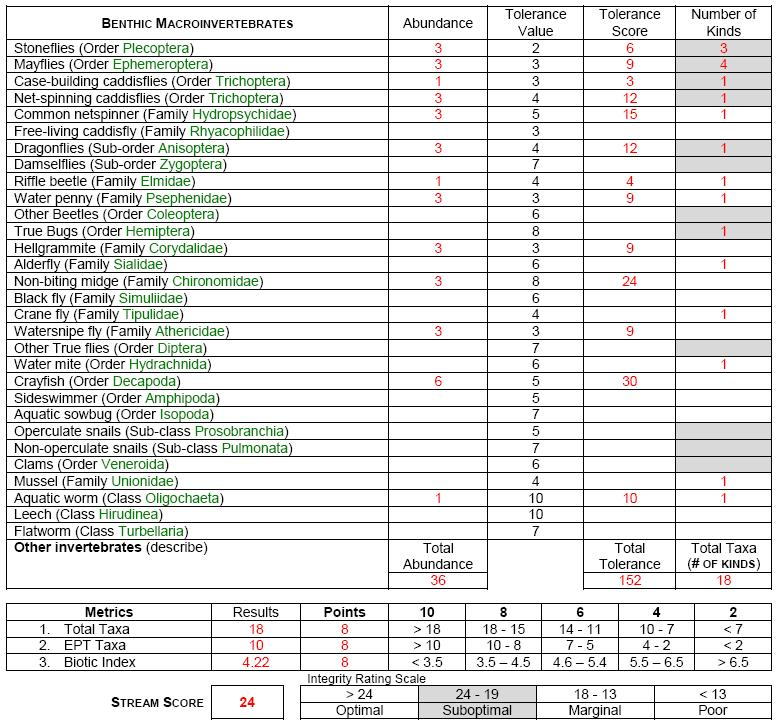# Biological integrity

 After the sorting and identification is complete, the macroinvertebrates are assessed using three different metrics that include two richness and one tolerance metric.  These metrics are integrated into an overall stream score, which is given an integrity rating.  Numbers can be calculated from abundance ratings by assigning a simple numbering system (A = 6; C = 3; R = 1).  The calculation procedure for each metric is described below: Total Taxa: This metric is simply the total number of different kinds from the entire sample. EPA Taxa: The total number of different kinds from the Ephemeroptera (mayfles), Plecoptera (stoneflies) and Trichoptera (caddisflies) orders.   Biotic Index: Multiply the abundance number by the tolerance rating to determine the tolerance score.  Add the entire tolerance score column and the abundance column.  Divide the total tolerance by the total abundance. Stream Score: The overall stream score is determined using a point scale system, which combines the metrics into a single number and integrity rating.​ Click-Here to view more advanced biological integrity metrics. Note: Spreadsheets that will calculate metrics and integrity ratings for all levels of surveys are available.